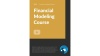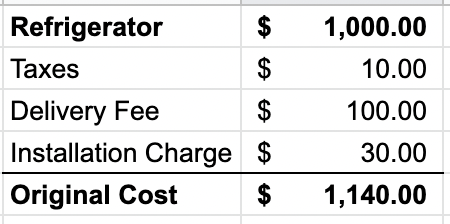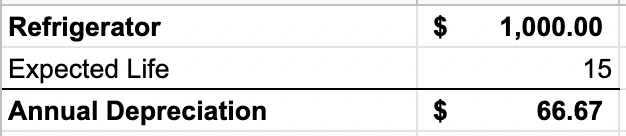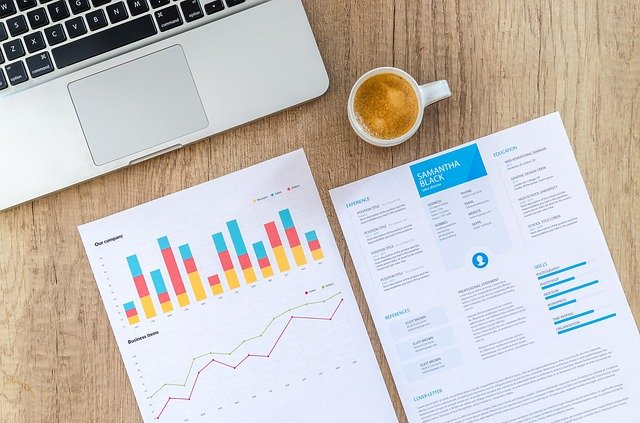# Net Book Value (NBV)

How are assets recorded by accountants?

Net book value (NBV) is the value of an asset at which it is recorded on the balance sheet after adjusting for accumulated non-cash charges such as depreciation, amortization, or depletion. NBV is usually calculated by reducing the asset's original purchase price by the accumulated non-cash charges.## Net Book Value: Formula

The net book value of an asset can be derived by using the following formula:

Net Book Value = Original Cost of Asset - Accumulated Non-Cash Charges

where,

• Original cost of asset: The price the asset was purchased for, plus all expenses and costs to bring the asset to use. These expenses and costs include: delivery fees, taxes, and installation.
• Accumulated non-cash charges: The total amount of depreciation, amortization, or depletion that the asset has incurred over its useful life.

We will go into further detail on how to calculate each variable below.### Calculating The Original Cost Of An Asset

Let's assume a restaurant purchased a new refrigerator (an asset) two years ago and would like to calculate the NBV of the refrigerator so that it may report it on its current balance sheet.

The cost of the refrigerator was \$1,000. The costs to bring the refrigerator to use include:

• Taxes: \$10
• Delivery: \$100
• Installation charge: \$30

The original cost of the asset for calculating NBV is \$1,140 (\$1,000 + \$10 + \$100 + \$30). This calculation is provided in the table below.### Non-Cash Charges: Depreciation, Amortization, Depletion

The second part of calculating NBV is to subtract non-cash charges that have accumulated over the asset's life.

Non-cash charges include:

• Depreciation: For a tangible asset, i.e. a refrigerator or car.
• Amortization: For an intangible asset, i.e. a patent or trademark.
• Depletion: Usually for raw materials or natural minerals, i.e., the amount of coal in a coal mine.

It is important to note that the net book value of an asset will depend on the depreciation method being utilized by the company. Two types of depreciation methods are straight-line depreciation and double-declining balance (accelerated depreciation).

Since a company may elect to use a straight-line or double-declining balance, the NBV of an asset may differ from the NBV of the same asset held by another company, holding all things, such as purchase date the asset's useful life, equal.

In order to arrive at accumulated depreciation, amortization, or depletion, the total amount of the non-cash charge must be netted out from the asset's original cost.Let's assume that the refrigerator, from our restaurant example, has a useful life of 15 years. For simplicity, let's also assume the restaurant is using straight-line depreciation. The annual depreciation of the refrigerator is \$66.67 (\$1,000 / 15 years).Let's now assume that the restaurant has had a refrigerator for a total of 5 years. The accumulated depreciation that the restaurant would report on its balance sheet is equal to \$333.33 [(1,000 / 5) x 5].## Calculating Net Book Value

Using the original cost of the refrigerator and the accumulated depreciation, we can now calculate the net book value the restaurant will record on its balance sheet.

The original cost of the refrigerator was \$1,140, and accumulated depreciation over five years was \$333.33.

NBV can now be calculated by subtracting the accumulated depreciation from the cost of the refrigerator and comes to \$806.67.

\$806.67 = \$1,140 - \$333.33### Everything You Need To Master Financial Statement Modeling

To Help you Thrive in the Most Prestigious Jobs on Wall Street.

## Net Book Value Vs. Market Value

Net book value and market value are two terms that both refer to the value of a company's assets; however, the value and use of each are different.

NBV refers to the original purchase price of an asset (including all fees and expenses) less accumulated depreciation, amortization, or depletion. It is often used by companies who want to record the asset's value on its financial statements or if the company is facing liquidation.

Market value is the price that an asset will sell for in the marketplace or the price a seller would pay the buyer for the asset. For example, equities (stocks) are bought and sold at their market value.

Since the market value of an asset depends on the supply and demand of the asset in the marketplace. Since net book value is calculated based on a historical value less depreciation, the asset's value won't be equal under both methods.## Net Book Value: Why It's Important

Net book value is significant because it allows a company to calculate its assets' value accurately. This helps investors understand the value of the underlying assets and how they have depreciated over time.

Companies use NBV for record-keeping. For example, it is beneficial if the company is facing liquidation; or if the company is merging or being sold to another company.

It is also used to compare the company's market value in ratio analysis. However, due to most companies trading at very high multiples of book value (after the popularization of the concept of discounted cash flow), this measure has lost its relevance.### Everything You Need To Build Your Accounting Skills

To Help you Thrive in the Most Flexible Job in the World.